Prev Next

# Chapter 5. Plugins, Fits, and Filters

## Using Plugins, Fits, and Filters

Many of the mathematical data operators in Kst, including fits and filters, are implemented as plugins. Plugins are loaded at run time and use a stable API, so it is possible to write your own plugins and include them in your local installation without re-compiling Kst. Fits and Filters are simply subsets of the set of plugins, and thus behave identically to generic plugins, with the exception of additional convenience dialog functionality when selected from the plot context menu. See here for a general description of the creation of fits and filters.

To date, there are more than 25 built-in plugins available in Kst that perform functions from taking cross correlations of two vectors to producing periodograms of a data set. The following screenshot shows the settings window for a typical plugin, created by selecting the desired plugin from the Standard Plugin, Fit Plugin or Filter Plugin submenus in the Create toolbar menu.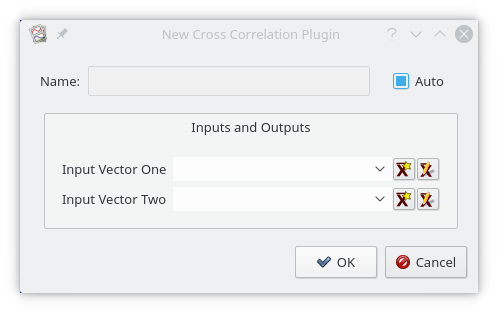The following sections describe the purpose, key algorithms or formulas used to perform calculations, and inputs and outputs for each plugin. Note that fitting and filtering plugins are included in the following sections.

### Autocorrelation

The autocorrelation plugin calculates correlation values between a series (vector) and a lagged version of itself, using lag values from `floor(-(N-1)/2)` to `floor((N-1)/2)`, where `N` is the number of points in the data set. The time vector is not an input as it is assumed that the data is sampled at equal time intervals. The correlation value `r` at lag `k` is: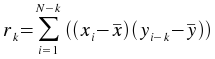#### Inputs

X Array (vector)

The array `x` of values to calculate correlation values for.

#### Outputs

Step Number (vector)

The array of step, or lag values.

Correlation Value (vector)

The array of correlation values calculated using the corresponding step number in the Step Number vector.

### Bin

The bin plugin bins elements of a single data vector into bins of a specified size. The value of each bin is the mean of the elements belonging to the bin. For example, if the bin size is `3`, and the input vector is `[9,2,7,3,4,74,5,322,444,2,1]`, then the outputted bins would be `[6,27,257]`. Note that any elements remaining at the end of the input vector that do not form a complete bin (in this case, elements `2` and `1`), are simply discarded.

#### Inputs

Input Vector (vector)

The vector to bin.

Bin Size (scalar)

The size to use for each bin.

#### Outputs

Bins (vector)

The array of means for each bin.

### Butterworth band-pass

The Butterworth band-pass plugin filters a set of data by calculating the Fourier transform of the data and recalculating the the frequency responses using the following formula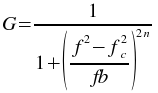where `f` is the frequency, `fc` is the low frequency cutoff, `b` is the bandwidth of the band to pass, and `n` is the order of the Butterworth filter. The inverse Fourier transform is then calculated using the new filtered frequency responses.

#### Inputs

X Array (vector)

The array of values to filter.

Order (scalar)

The order of the Butterworth filter to use.

Low cutoff frequency (scalar)

The low cutoff frequency of the Butterworth band pass filter.

Band width (scalar)

The width of the band to pass. This should be the difference between the desired high cutoff frequency and the low cutoff frequency.

#### Outputs

X Filtered (vector)

The array of filtered data values.

### Butterworth band-stop

The Butterworth band-stop plugin filters a set of data by calculating the Fourier transform of the data and recalculating the the frequency responses using the following formula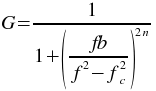where `f` is the frequency, `fc` is the low frequency cutoff, `b` is the bandwidth of the band to stop, and `n` is the order of the Butterworth filter. The inverse Fourier transform is then calculated using the new filtered frequency responses.

#### Inputs

X Array (vector)

The array of values to filter.

Order (scalar)

The order of the Butterworth filter to use.

Low cutoff frequency (scalar)

The low cutoff frequency of the Butterworth band stop filter.

Band width (scalar)

The width of the band to stop. This should be the difference between the desired high cutoff frequency and the low cutoff frequency.

#### Outputs

X Filtered (vector)

The array of filtered data values.

### Butterworth high-pass

The Butterworth high-pass plugin filters a set of data by calculating the Fourier transform of the data and recalculating the the frequency responses using the following formulawhere `f` is the frequency, `fc` is the high frequency cutoff, and `n` is the order of the Butterworth filter. The inverse Fourier transform is then calculated using the new filtered frequency responses.

#### Inputs

X Array (vector)

The array of values to filter.

Order (scalar)

The order of the Butterworth filter to use.

Cutoff frequency (scalar)

The cutoff frequency of the Butterworth high pass filter.

#### Outputs

X Filtered (vector)

The array of filtered data values.

### Butterworth low-pass

The Butterworth low-pass plugin filters a set of data by calculating the Fourier transform of the data and recalculating the the frequency responses using the following formula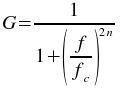where `f` is the frequency, `fc` is the low frequency cutoff, and `n` is the order of the Butterworth filter. The inverse Fourier transform is then calculated using the new filtered frequency responses.

#### Inputs

X Array (vector)

The array of values to filter.

Order (scalar)

The order of the Butterworth filter to use.

Cutoff frequency (scalar)

The cutoff frequency of the Butterworth low pass filter.

#### Outputs

X Filtered (vector)

The array of filtered data values.

### Chop

The chop plugin takes an input vector and divides it into two vectors. Every second element in the input vector is placed in one output vector, while all other elements from the input vector are placed in another output vector.

#### Inputs

Array (vector)

The array of values to perform the chop on.

#### Outputs

Odd Array (vector)

The array containing the odd part of the input array (i.e. it contains the first element of the input array).

Even Array (vector)

The array containing the even part of the input array (i.e. it does not contain the first element of the input array).

Difference Array (vector)

The array containing the elements of the odd array minus the respective elements of the even array.

Index Array (vector)

An index array the same length as the other three output arrays.

### Convolution

The convolution plugin generates the convolution of one vector with another. The convolution of two functions `f` and `g` is given by: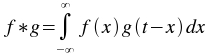The order of the vectors does not matter, since `f*g=g*f`. In addition, the vectors do not need to be of the same size, as the plugin will automatically extrapolate the smaller vector.

#### Inputs

Array One (vector)

One of the pair of arrays to take the convolution of.

Array Two (vector)

One of the pair of arrays to take the convolution of.

#### Outputs

Convolved (vector)

The convolution of the two input vectors.

### Crosscorrelation

The crosscorrelation plugin calculates correlation values between two series (vectors) `x` and `y`, using lag values from `floor(-(N-1)/2)` to `floor((N-1)/2)`, where `N` is the number of elements in the longer vector. The shorter vector is padded to the length of the longer vector using `0`s. The time vector is not an input as it is assumed that the data is sampled at equal time intervals. The correlation value `r` at lag `k` is: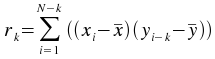#### Inputs

X Array (vector)

The array `x` used in the cross-correlation formula.

Y Array (vector)

The array `y` used in the cross-correlation formula.

#### Outputs

Step Number (vector)

The array of step, or lag values.

Correlation Value (vector)

The array of correlation values calculated using the corresponding step number in the Step Number vector.

### Deconvolution

The deconvolution plugin generates the deconvolution of one vector with another. Deconvolution is the inverse of convolution. Given the convolved vector `h` and another vector `g`, the deconvolution `f` is given by: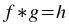The vectors do not need to be of the same size, as the plugin will automatically extrapolate the shorter vector. The shorter vector is assumed to be the response function `g`.

#### Inputs

Array One (vector)

One of the pair of arrays to take the deconvolution of.

Array Two (vector)

One of the pair of arrays to take the deconvolution of.

#### Outputs

Deconvolved (vector)

The deconvolution of the two input vectors.

### Fit exponential weighted

The Fit exponential weighted plugin performs a weighted non-linear least-squares fit to an exponential model: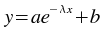An initial estimate of `a=1.0`,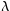`=0`, and `b=0` is used. The plugin subsequently iterates to the solution until a precision of `1.0e-4` is reached or 500 iterations have been performed.

#### Inputs

X Array (vector)

The array of x values for the data points to be fitted.

Y Array (vector)

The array of y values for the data points to be fitted.

Weights (vector)

The array of weights to use for the fit.

#### Outputs

Y Fitted (vector)

The array of fitted y values.

Residuals (vector)

The array of residuals.

Parameters (vector)

The best fit parameters `a`,, and `b`.

Covariance (vector)

The covariance matrix of the model parameters, returned row after row in the vector.

chi^2/nu (scalar)

The weighted sum of squares of the residuals, divided by the degrees of freedom.

### Fit exponential

The Fit exponential plugin is identical in function to the Fit exponential weighted plugin with the exception that the weight value `wi` is equal to `1` for all index values `i`. As a result, the Weights (vector) input does not exist.

### Fit gaussian weighted

The Fit gaussian weighted plugin performs a weighted non-linear least-squares fit to a Gaussian model: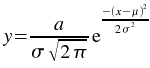An initial estimate of `a=`(maximum of the y values),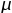`=`(mean of the x values), and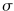`=`(the midpoint of the x values) is used. The plugin subsequently iterates to the solution until a precision of `1.0e-4` is reached or 500 iterations have been performed.

#### Inputs

X Array (vector)

The array of x values for the data points to be fitted.

Y Array (vector)

The array of y values for the data points to be fitted.

Weights (vector)

The array of weights to use for the fit.

#### Outputs

Y Fitted (vector)

The array of fitted y values.

Residuals (vector)

The array of residuals.

Parameters (vector)

The best fit parameters,, and `a`.

Covariance (vector)

The covariance matrix of the model parameters, returned row after row in the vector.

chi^2/nu (scalar)

The weighted sum of squares of the residuals, divided by the degrees of freedom.

### Fit gaussian

The Fit gaussian plugin is identical in function to the Fit gaussian weighted plugin with the exception that the weight value `wi` is equal to `1` for all index values `i`. As a result, the Weights (vector) input does not exist.

The gradient weighted plugin performs a weighted least-squares fit to a straight line model without a constant term: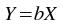The best-fit is found by minimizing the weighted sum of squared residuals: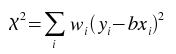for `b`, where `wi` is the weight at index `i`.

#### Inputs

X Array (vector)

The array of x values for the data points to be fitted.

Y Array (vector)

The array of y values for the data points to be fitted.

Weights (vector)

The array containing weights to be used for the fit.

#### Outputs

Y Fitted (vector)

The array of y values for the points representing the best-fit line.

Residuals (vector)

The array of residuals, or differences between the y values of the best-fit line and the y values of the data points.

Parameters (vector)

The parameter `b` of the best-fit.

Covariance (vector)

The estimated covariance matrix, returned row after row, starting with row 0.

Y Lo (vector)

The corresponding value in Y Fitted minus the standard deviation of the best-fit function at the corresponding x value.

Y Hi (vector)

The corresponding value in Y Fitted plus the standard deviation of the best-fit function at the corresponding x value.

chi^2/nu (scalar)

The value of the sum of squares of the residuals, divided by the degrees of freedom.

The Fit linear plugin is identical in function to the Fit gradient weighted plugin with the exception that the weight value `wi` is equal to `1` for all index values `i`. As a result, the Weights (vector) input does not exist.

### Fit linear weighted

The Fit linear weighted plugin performs a weighted least-squares fit to a straight line model: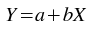The best-fit is found by minimizing the weighted sum of squared residuals: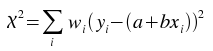for `a` and `b`, where `wi` is the weight at index `i`.

#### Inputs

X Array (vector)

The array of x values for the data points to be fitted.

Y Array (vector)

The array of y values for the data points to be fitted.

Weights (vector)

The array containing weights to be used for the fit.

#### Outputs

Y Fitted (vector)

The array of y values for the points representing the best-fit line.

Residuals (vector)

The array of residuals, or differences between the y values of the best-fit line and the y values of the data points.

Parameters (vector)

The parameters `a` and `b` of the best-fit.

Covariance (vector)

The estimated covariance matrix, returned row after row, starting with row 0.

Y Lo (vector)

The corresponding value in Y Fitted minus the standard deviation of the best-fit function at the corresponding x value.

Y Hi (vector)

The corresponding value in Y Fitted plus the standard deviation of the best-fit function at the corresponding x value.

chi^2/nu (scalar)

The value of the sum of squares of the residuals, divided by the degrees of freedom.

### Fit linear

The Fit linear plugin is identical in function to the Fit linear weighted plugin with the exception that the weight value `wi` is equal to `1` for all index values `i`. As a result, the Weights (vector) input does not exist.

### Fit lorentzian weighted

The Fit lorentzian weighted plugin performs a weighted non-linear least-squares fit to a Lorentzian model: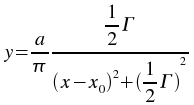An initial estimate of `a=`(maximum of the y values), `x0=`(mean of the x values), and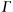`=`(the midpoint of the x values) is used. The plugin subsequently iterates to the solution until a precision of `1.0e-4` is reached or 500 iterations have been performed.

#### Inputs

X Array (vector)

The array of x values for the data points to be fitted.

Y Array (vector)

The array of y values for the data points to be fitted.

Weights (vector)

The array of weights to use for the fit.

#### Outputs

Y Fitted (vector)

The array of fitted y values.

Residuals (vector)

The array of residuals.

Parameters (vector)

The best fit parameters `x0`,, and `a`.

Covariance (vector)

The covariance matrix of the model parameters, returned row after row in the vector.

chi^2/nu (scalar)

The weighted sum of squares of the residuals, divided by the degrees of freedom.

### Fit lorentzian

The Fit lorentzian plugin is identical in function to the Fit lorentzian weighted plugin with the exception that the weight value `wi` is equal to `1` for all index values `i`. As a result, the Weights (vector) input does not exist.

### Fit polynomial weighted

The Fit polynomial weighted plugin performs a weighted least-squares fit to a polynomial model: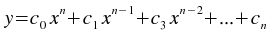where `n` is the degree of the polynomial model.

#### Inputs

X Array (vector)

The array of x values for the data points to be fitted.

Y Array (vector)

The array of y values for the data points to be fitted.

Weights (vector)

The array of weights to use for the fit.

Order (scalar)

The order, or degree, of the polynomial model to use.

#### Outputs

Y Fitted (vector)

The array of fitted y values.

Residuals (vector)

The array of residuals.

Parameters (vector)

The best fit parameters `c0`, `c1`,..., `cn`.

Covariance (vector)

The covariance matrix of the model parameters, returned row after row in the vector.

chi^2/nu (scalar)

The weighted sum of squares of the residuals, divided by the degrees of freedom.

### Fit polynomial

The Fit polynomial plugin is identical in function to the Fit polynomial weighted plugin with the exception that the weight value `wi` is equal to `1` for all index values `i`. As a result, the Weights (vector) input does not exist.

### Fit sinusoid weighted

The Fit sinusoid weighted plugin performs a least-squares fit to a sinusoid model: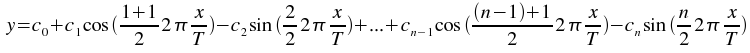where `T` is the specified period, and `n=2+2H`, where `H` is the specified number of harmonics.

#### Inputs

X Array (vector)

The array of x values for the data points to be fitted.

Y Array (vector)

The array of y values for the data points to be fitted.

Weights (vector)

The array of weights to use for the fit.

Harmonics (scalar)

The number of harmonics of the sinusoid to fit.

Period (scalar)

The period of the sinusoid to fit.

#### Outputs

Y Fitted (vector)

The array of fitted y values.

Residuals (vector)

The array of residuals.

Parameters (vector)

The best fit parameters `c0`, `c1`,..., `cn`.

Covariance (vector)

The covariance matrix of the model parameters, returned row after row in the vector.

chi^2/nu (scalar)

The weighted sum of squares of the residuals, divided by the degrees of freedom.

### Fit sinusoid

The Fit sinusoid plugin is identical in function to the Fit sinusoid weighted plugin with the exception that the weight value `wi` is equal to `1` for all index values `i`. As a result, the Weights (vector) input does not exist.

### Interpolation Akima spline

The Interpolation Akima spline plugin generates a non-rounded Akima spline interpolation for the supplied data set, using natural boundary conditions.

#### Inputs

X Array (vector)

The array of x values of the data points to generate the interpolation for.

Y Array (vector)

The array of y values of the data points to generate the interpolation for.

X' Array (vector)

The array of x values for which interpolated y values are desired.

#### Outputs

Y Interpolated (vector)

The interpolated y values.

### Interpolation Akima spline periodic

The kstinterp akima periodic plugin generates a non-rounded Akima spline interpolation for the supplied data set, using periodic boundary conditions.

#### Inputs

X Array (vector)

The array of x values of the data points to generate the interpolation for.

Y Array (vector)

The array of y values of the data points to generate the interpolation for.

X' Array (vector)

The array of x values for which interpolated y values are desired.

#### Outputs

Y Interpolated (vector)

The interpolated y values.

### Interpolation cubic spline

The Interpolation cubic spline plugin generates a cubic spline interpolation for the supplied data set, using natural boundary conditions.

#### Inputs

X Array (vector)

The array of x values of the data points to generate the interpolation for.

Y Array (vector)

The array of y values of the data points to generate the interpolation for.

X' Array (vector)

The array of x values for which interpolated y values are desired.

#### Outputs

Y Interpolated (vector)

The interpolated y values.

### Interpolation cubic spline periodic

The Interpolation cubic spline periodic plugin generates a cubic spline interpolation for the supplied data set, using periodic boundary conditions.

#### Inputs

X Array (vector)

The array of x values of the data points to generate the interpolation for.

Y Array (vector)

The array of y values of the data points to generate the interpolation for.

X' Array (vector)

The array of x values for which interpolated y values are desired.

#### Outputs

Y Interpolated (vector)

The interpolated y values.

### Interpolation linear

The Interpolation linear plugin generates a linear interpolation for the supplied data set.

#### Inputs

X Array (vector)

The array of x values of the data points to generate the interpolation for.

Y Array (vector)

The array of y values of the data points to generate the interpolation for.

X' Array (vector)

The array of x values for which interpolated y values are desired.

#### Outputs

Y Interpolated (vector)

The interpolated y values.

### Interpolation polynomial

The Interpolation polynomial plugin generates a polynomial interpolation for the supplied data set. The number of terms in the polynomial used is equal to the number of points in the supplied data set.

#### Inputs

X Array (vector)

The array of x values of the data points to generate the interpolation for.

Y Array (vector)

The array of y values of the data points to generate the interpolation for.

X' Array (vector)

The array of x values for which interpolated y values are desired.

#### Outputs

Y Interpolated (vector)

The interpolated y values.

The Noise addition plugin adds a Gaussian random variable to each element of the input vector. The Gaussian distribution used has a mean of `0` and the specified standard deviation. The probability density function of a Gaussian random variable is: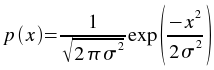#### Inputs

Array (vector)

The array of elements to which random noise is to be added.

Sigma (scalar)

The standard deviation to use for the Gaussian distribution.

#### Outputs

Output Array (vector)

The array of elements with Gaussian noise added.

### Periodogram

The periodogram plugin produces the periodogram of a given data set. One of two algorithms is used depending on the size of the data set—a fast algorithm is used if there are greater than 100 data points, while a slower algorithm is used if there are less than or equal to 100 data points.

#### Inputs

Time Array (vector)

The array of time values of the data points to generate the interpolation for.

Data Array (vector)

The array of data values, dependent on the time values, of the data points to generate the interpolation for.

Oversampling factor (scalar)

The factor to oversample by.

Average Nyquist frequency factor (scalar)

The average Nyquist frequency factor.

#### Outputs

Frequency (vector)

The frequency vector.

Periodogram (vector)

The frequency response vector for the periodogram.

### Statistics

The statistics plugin calculates statistics for a given data set. Most of the output scalars are named such that the values they represent should be apparent. Standard formulas are used to calculate the statistical values.

#### Inputs

Data Array (vector)

The array of data values to calculate statistics for.

#### Outputs

Mean (scalar)

The mean of the data values.

Minimum (scalar)

The minimum value found in the data array.

Maximum (scalar)

The maximum value found in the data array.

Variance (scalar)

The variance of the data set.

Standard deviation (scalar)

The standard deviation of the data set.

Median (scalar)

The median of the data set.

Absolute deviation (scalar)

The absolute deviation of the data set.

Skewness (scalar)

The skewness of the data set.

Kurtosis (scalar)

The kurtosis of the data set.

 Prev Contents Next Exporting Tabs Up Licensing